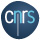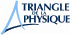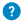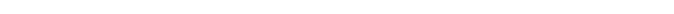Phase transition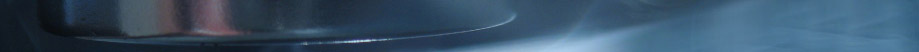Phase transition

To be or not to be superconducting

When a superconductor is heated, it stops being a superconductor at a very precise temperature called the critical temperature Tc. We call this phase transition, like when water transforms into ice at precisely 0° C. The Russian physicists Landau described superconductors (long before the causes of superconductivity were discovered) in a way that helps understanding this phase transition. Indeed, this description does not explain where the condensate comes from, but it describes the way it behaves when the temperature of the material is changed. In this “phenomenological” approach, Landau assumes that the condensate does exist and that he could describe it with a quantum wavefunction, characterized by an amplitude and a phase. Landau proposed that a superconductor is a more “orderly” environment than a non superconducting environment, and that the wavefunction could describe this new order.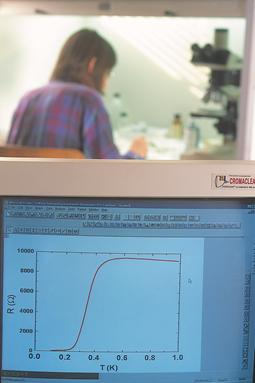Landau then intuited the equations ruling this wavefunction. These equations help us understand the effects of the magnetic field or of the temperature. This belongs to the framework called “thermodynamic” by physicists. These equations also predict the existence of vortices. This model enables us to predict the behaviours of all the parameters characterizing superconductors depending on temperature, especially close to the critical temperature Tc.

Since this model does not explain why the condensate exists but only assumes it does, it can be used to explain the properties of new superconductors such as cuprates even though the origin of this type of superconductivity is not well known. This kind of model can also be used with magnets and superfluids.